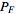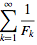Categories

# Python II: Fibonacci Sequence

Tim’s second exercise (here’s the first):

Write a recursive function that calculates the value of Fibonacci numbers. These are your acceptance criteria:

• Calculating fib(250) must return 7896325826131730509282738943634332893686268675876375
• The function must use recursion. No intermediary data structures, etc.
• The implementation must be written in pure python – no C extension modules, that’s cheating.
• The function must calculate the 250th Fibonacci number in under one second.

You will get extra points if:

• You can also demonstrate a proof for the Reciprocal Fibonacci constant, meeting the following conditions
• Your proof must also run in under one second
• Your proof must not duplicate any of the concerns addressed by your original Fibonacci function implementation.
• Your proof is allowed to call into the Fibonacci function though!

The Reciprocal Fibonacci constant is defined as:== 3.35988566…

Where F is a Fibonacci number

Here’s a couple of hints about things to look for:

• Memoization
• Function decorators

I haven’t tried the extra credit section yet, but this is what I came up with. Again, starting with a naive solution with some doctest tests:

```#!/usr/bin/env python

"""
>>> fib(1)
1
>>> fib(2)
1
>>> fib(3)
2
>>> fib(4)
3
>>> fib(5)
5
"""
def fib(n):
if n <= 2:
return 1
else:
return fib(n-2) + fib(n-1)

def _test():
import doctest
doctest.testmod()

if __name__ == "__main__":
_test()
```

Well that seems to work OK. Now, try adding a test for the 250th number:

```>>> fib(250)
7896325826131730509282738943634332893686268675876375
```

And wait … and wait …

No, doesn't look like that's going to return any time soon. Of course when you look at what it's doing, the complexity's increasing in the order of 2n or something, which isn't good.

OK, I guess this is where memoisation comes in. After much head-scratching on the train this morning, I came up with this:

```fibs = {}

def fib(n):
if n <= 2:
return 1
elif fibs.has_key(n):
return fibs[n]
else:
fibs[n] = fib(n-1) + fib(n-2)
return fibs[n]
```

A little tweak to allow it to be called from the command line with the value of n passed as an argument, and here's the final version:

```#!/usr/bin/env python
import sys

fibs = {}

def fib(n):
if n <= 2:
return 1
elif fibs.has_key(n):
return fibs[n]
else:
fibs[n] = fib(n-1) + fib(n-2)
return fibs[n]

def _test():
import doctest
doctest.testmod()

if len(sys.argv) > 1:
print fib(int(sys.argv))
if __name__ == "__main__":
_test()
```
```fib(master) \$ time ./fib.py 250
7896325826131730509282738943634332893686268675876375

real	0m0.280s
user	0m0.161s
sys	0m0.047s
```

Sorted!

[tags]python, web21c, fibonacci[/tags]

## One reply on “Python II: Fibonacci Sequence”

Nice work. That’s what I’m looking for. Now if you can just do the same thing with the bonus part, without duplicating the caching code in that function…